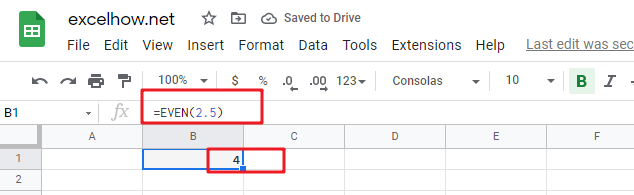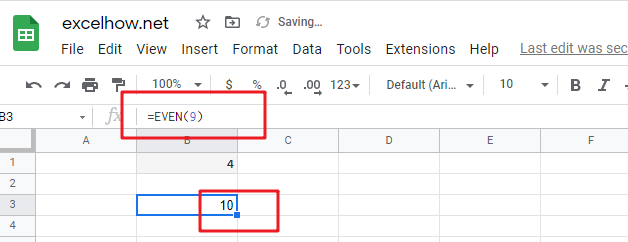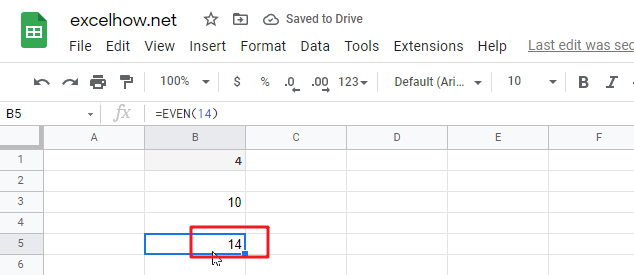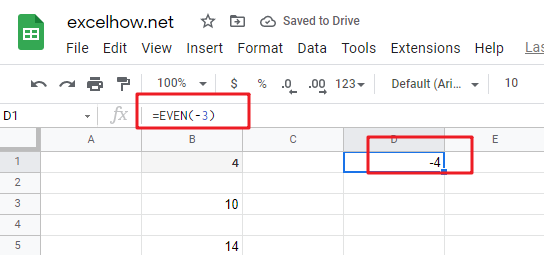# ExcelHow

This post will guide you how to use Google Sheets EVEN function with syntax and examples.

## Description

The Google Sheets EVEN function rounds a given number up to the nearest even integer.

You can use the EVEN function to return the next even integer after rounding a supplied number up in google sheets. The returned value is away from zero. It means that if the number is a positive value and the return value will become larger and if the number is a negative value, the return value will become smaller.

The EVEN function is a build-in function in Google Sheets and it is categorized as a MATH function.

## Syntax

he syntax of the EVEN function is as below:

=EVEN (number)

Where the EVEN function arguments is:

• number -This is a required argument. . A numeric value that you want to round up to the nearest even integer.

Note:

• If the number argument is not numeric value, the EVEN function will return the #VALUE! Error.

## Google Sheets EVEN Function Examples

The below examples will show you how to use google sheets EVEN Function to round up a given number to the nearest even integer.

1# to round 2.5 up to the nearest even integer, enter the following formula in Cell B1.

`=EVEN(2.5)`2# to round 9 to the nearest even integer, enter the following formula in Cell B2.

`=EVEN(9)`3# to round 14 up to the nearest even integer, enter the following formula in Cell B3.

`=EVEN(14)`4# to round -3 up to the nearest even integer, enter the following formula in Cell B4.

`=EVEN(-3)`